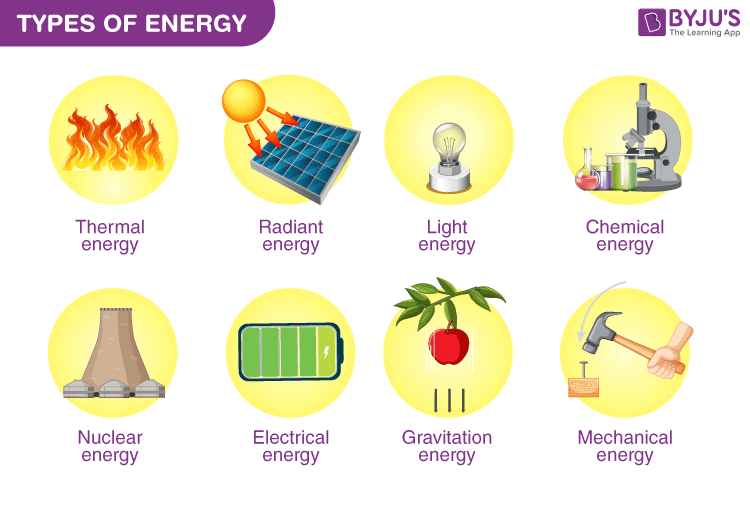# Types of Energy

You have heard this a hundred times from your teacher, but have you ever asked what kind of work has to be accomplished to define energy? Work is said to be done when an object is moved against a force, like gravity.

## What Is Energy?

Energy is a scalar quantity whose SI unit is the joule (J). Energy is found in many things, and thus there are different types of energy. All forms of energy are either kinetic or potential.### Kinetic Energy:

The energy in motion is known as Kinetic Energy. For example, a moving ball, flowing water etc.

$$\begin{array}{l}Kinetic\; Energy =\frac{1}{2}m\times v^{2}\end{array}$$

Where,

m = Mass of the object

v = Velocity of the object

Read More: Different Types of Energy

### Potential Energy:

This is the energy stored in an object and is measured by the amount of work done. For example: a pen on a table, water in a lake etc.

$$\begin{array}{l}Potential\; Energy =m\times g\times h\end{array}$$

Where,

m = Mass of the object (in kilograms)

g = Acceleration due to gravity

h = Height in meters

Various other forms of energy are as follows –

### Mechanical Energy:

It is the sum of potential energy and kinetic energy that is the energy associated with the motion & the position of an object, known as Mechanical energy. Thus, we can derive the formula of mechanical energy as –

Mechanical Energy = Kinetic Energy + Potential Energy

$$\begin{array}{l}Mechanical\; Energy= \frac{1}{2}mv^{2}+mgh\end{array}$$

### Chemical Energy:

Chemical energy is stored in a chemical substance in between the bonds of atoms in compounds. In the same given temperature and environment, energy can be released (or absorbed) because a reaction between a set of chemical substances equals the difference between the energy content of the products and the reactants.

Some other types of energy are Electric, Magnetic, Radiant, Nuclear, Ionization, Elastic, Gravitational, Thermal, Heat & Mechanical work.

### Different Types of Energy Resources:

Energy sources are used as fuels. Fuels are used in machinery, automobiles, and other industries. They are used in thermal plants to generate electricity. Energy resources can be classified into two types – Non-renewable and Renewable.

#### Non-renewable Resources:

Fossil fuels like oil, natural gas and coal are known as non-renewable resources, because once used, they cannot be renewed by natural process or means.

#### Renewable Resources:

Natural resources like wind, water, solar, and geothermal are called renewable resources as they come from sources that regenerate them back after consumption and are continuously available in nature.

Types of energy based on their resources are :

• Bio-energy
• Coal
• Geothermal
• Hydrogen
• Hydro-power
• Natural Gas
• Nuclear
• Oil
• Solar
• Wind
• Wood

## What are Kinetic Energy and Potential Energy?## Frequently Asked Questions – FAQs

Q1

### What is energy?

Energy is the ability to do work.
Q2

### Energy is a scalar or a vector quantity?

Energy is a scalar quantity.
Q3

### What is the unit of energy?

The SI unit of energy is the joule.
Q4

### What is kinetic energy?

Kinetic energy is the energy associated with the object’s motion. Example: moving ball.
Q5

### How are energy resources classified?

Energy resources are classified into:
• Non-renewable Resources
• Renewable Resources